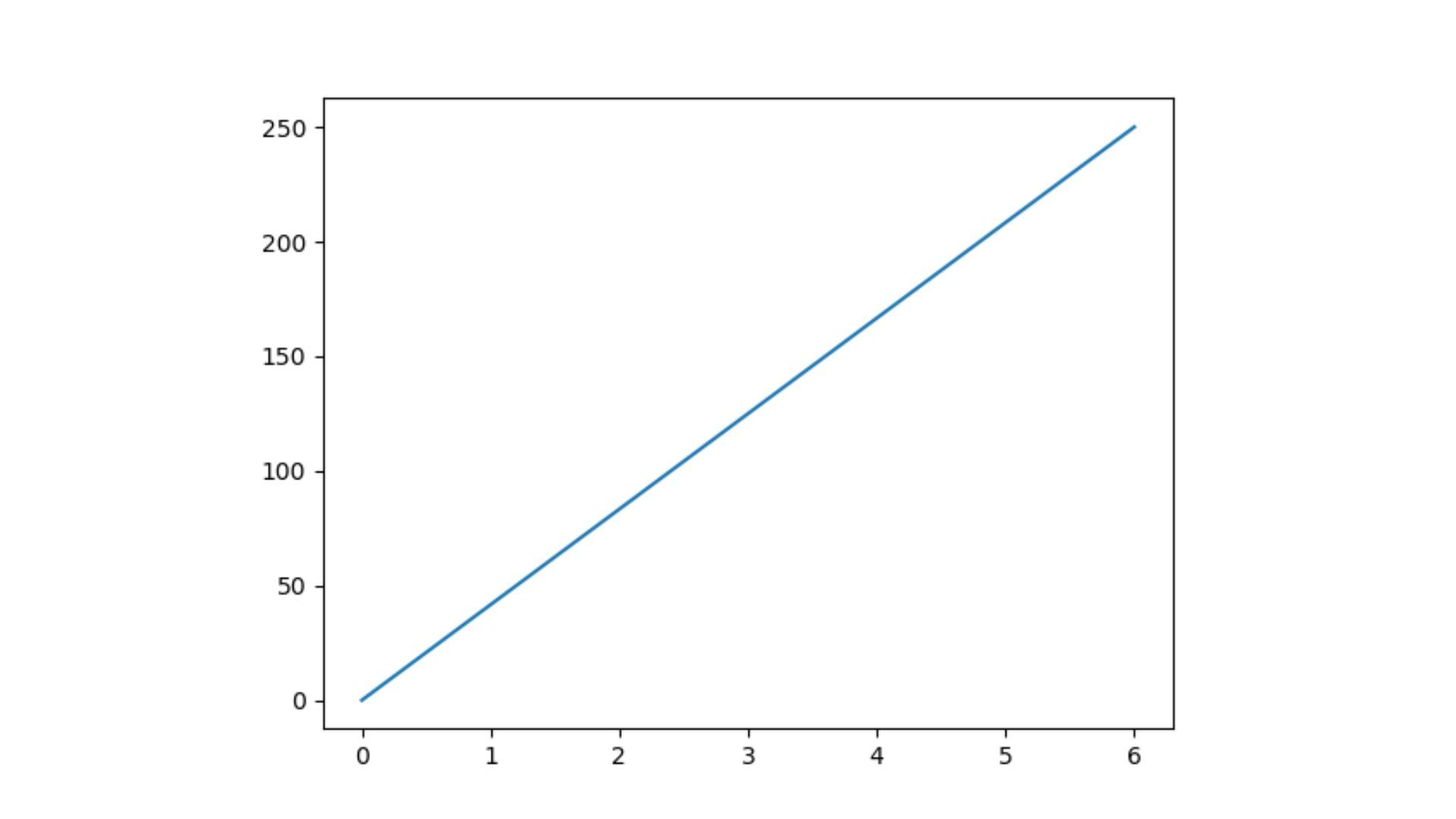# How to Plot in Python using Pyplot?## Introduction

Matplotlib is the package used for data visualization and is one of the most popular packages in python. As the name suggests Matplotlib works like MATLAB. Pyplot is a cluster of functions that makes matplotlib like MATLAB.

Each pyplot function takes is used to make some changes to a figure e.g., plotting some line in an area, decorating the plot with labels, creating a figure, etc.

##### Creating visualizations with pyplot is quite easy
``````import matplotlib.pyplot as plt
plt.plot([5, 4, 3, 2 1])
plt.ylabel('tax rate')
plt.show()
``````

## Matplotlib.pyplot.legend()

A legend is used to describe elements for a particular area of a graph. Python has a function called legend() which is used to place a legend on the axis.

The legend function has an attribute called loc which is used to denote the location of the legend. The default value of attribute loc is upper left. Other string, such as upper right, lower left, lower right can also be used.

Legend can also be placed at a particular index also there is an attribute named bbox_to_anchor which takes a coordinate (x, y) as input and places the legend at the particular coordinate another attribute ncol can be used to denote the number of columns in the legend.

A few more attributes of legend function are:

1. fontsize: This is used to denote the font of the legend. It has a numeric value.
2. facecolor: This is used to denote the legend’s background color.
3. edgecolor: This is used to denote the legends patch edge color.
4. shadow: It is a boolean variable to denote whether to display shadow behind the legend or not.
5. title: This attribute denotes the legend’s title.
6. numpoints: The number of marker points in the legend when creating an entry for a Line2D (line).

## Examples

1. Simple legend

``````import numpy as np
import matplotlib.pyplot as plt

#X axis points
x = [-2, 2, 5, 9, 15]

#Y axis points
y = [-4, -2, 0, 1, 24]

#To plot the function
plt.plot(x, y)

#Function to create a legend
plt.legend(['fluctuation rate'])
plt.show()

``````
write your code here: Coding Playground

2. Changing the position of the legend

``````import numpy as np
import matplotlib.pyplot as plt

#values to plot
y1 = [-1, 0, 2.5]
y2 = [1, 1.5, 5]

plt.plot(y1)
plt.plot(y2)

#specifying location of the legend
plt.legend(["blue", "orange"], loc ="lower right")
plt.show()

``````

3. Plotting legend outside graph area

``````import numpy as np
import matplotlib.pyplot as plt

# Y-axis values
y1 = [0, 2, 4, 6, 8, 10, 12, 14, 16]
# Y-axis values
y2 = [0, 1, 2, 3, 4, 5, 6, 7, 8]

# Function to plot coordinates
plt.plot(y1, label = "y = x")
plt.plot(y2, label = "y = 2x")

# Function to add a legend
plt.legend(bbox_to_anchor =(0.75, 1.15), ncol = 2)
plt.show()

``````